# Capacitive Reactance

## AC Electric Circuits

• #### Question 1

 Don’t just sit there! Build something!!

Learning to mathematically analyze circuits requires much study and practice. Typically, students practice by working through lots of sample problems and checking their answers against those provided by the textbook or the instructor. While this is good, there is a much better way.

You will learn much more by actually building and analyzing real circuits, letting your test equipment provide the “answers” instead of a book or another person. For successful circuit-building exercises, follow these steps:

1. Carefully measure and record all component values prior to circuit construction.
2. Draw the schematic diagram for the circuit to be analyzed.
3. Carefully build this circuit on a breadboard or other convenient medium.
4. Check the accuracy of the circuit’s construction, following each wire to each connection point, and verifying these elements one-by-one on the diagram.
5. Mathematically analyze the circuit, solving for all voltage and current values.
6. Carefully measure all voltages and currents, to verify the accuracy of your analysis.
7. If there are any substantial errors (greater than a few percent), carefully check your circuit’s construction against the diagram, then carefully re-calculate the values and re-measure.

For AC circuits where inductive and capacitive reactances (impedances) are a significant element in the calculations, I recommend high quality (high-Q) inductors and capacitors, and powering your circuit with low frequency voltage (power-line frequency works well) to minimize parasitic effects. If you are on a restricted budget, I have found that inexpensive electronic musical keyboards serve well as “function generators” for producing a wide range of audio-frequency AC signals. Be sure to choose a keyboard “voice” that closely mimics a sine wave (the “panflute” voice is typically good), if sinusoidal waveforms are an important assumption in your calculations.

As usual, avoid very high and very low resistor values, to avoid measurement errors caused by meter “loading”. I recommend resistor values between 1 kΩ and 100 kΩ.

One way you can save time and reduce the possibility of error is to begin with a very simple circuit and incrementally add components to increase its complexity after each analysis, rather than building a whole new circuit for each practice problem. Another time-saving technique is to re-use the same components in a variety of different circuit configurations. This way, you won’t have to measure any component’s value more than once.

• #### Question 2

As a general rule, capacitors oppose change in (choose: voltage or current), and they do so by . . . (complete the sentence).

Based on this rule, determine how a capacitor would react to a constant AC voltage that increases in frequency. Would an capacitor pass more or less current, given a greater frequency? Explain your answer.

• #### Question 3

We know that the formula relating instantaneous voltage and current in a capacitor is this:

 i = C de dt

Knowing this, determine at what points on this sine wave plot for capacitor voltage is the capacitor current equal to zero, and where the current is at its positive and negative peaks. Then, connect these points to draw the waveform for capacitor current: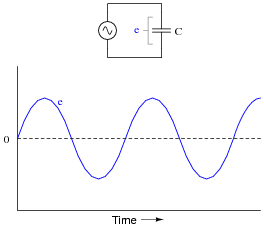How much phase shift (in degrees) is there between the voltage and current waveforms? Which waveform is leading and which waveform is lagging?

• #### Question 4

You should know that a capacitor is formed by two conductive plates separated by an electrically insulating material. As such, there is no “ohmic” path for electrons to flow between the plates. This may be vindicated by an ohmmeter measurement, which tells us a capacitor has (nearly) infinite resistance once it is charged to the ohmmeter’s full output voltage.

Explain then, how a capacitor is able to continuously pass alternating current, even though it cannot continuously pass DC.

• #### Question 5

Does a capacitor’s opposition to alternating current increase or decrease as the frequency of that current increases? Also, explain why we refer to this opposition of AC current in a capacitor as reactance instead of resistance.

• #### Question 6

Will the current through the resistor increase or decrease as the capacitor plates are moved closer together?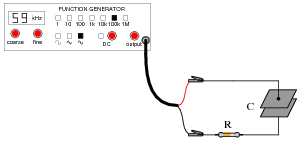Explain why this happens, with reference to capacitive reactance (XC).

• #### Question 7

Suppose someone were to ask you to differentiate electrical reactance (X) from electrical resistance (R). How would you distinguish these two similar concepts from one another, using your own words?

• #### Question 8

A capacitor rated at 2.2 microfarads is subjected to a sinusoidal AC voltage of 24 volts RMS, at a frequency of 60 hertz. Write the formula for calculating capacitive reactance (XC), and solve for current through the capacitor.

• #### Question 9

At what frequency does a 33 μF capacitor have 20 Ω of reactance? Write the formula for solving this, in addition to calculating the frequency.

• #### Question 10

Explain all the steps necessary to calculate the amount of current in this capacitive AC circuit: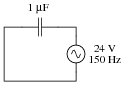• #### Question 11

In DC circuits, we have Ohm’s Law to relate voltage, current, and resistance together:

 E = I R

In AC circuits, we similarly need a formula to relate voltage, current, and impedance together. Write three equations, one solving for each of these three variables: a set of Ohm’s Law formulae for AC circuits. Be prepared to show how you may use algebra to manipulate one of these equations into the other two forms.

• #### Question 12

It is often necessary to represent AC circuit quantities as complex numbers rather than as scalar numbers, because both magnitude and phase angle are necessary to consider in certain calculations.

When representing AC voltages and currents in polar form, the angle given refers to the phase shift between the given voltage or current, and a “reference” voltage or current at the same frequency somewhere else in the circuit. So, a voltage of 3.5 V ∠−45o means a voltage of 3.5 volts magnitude, phase-shifted 45 degrees behind (lagging) the reference voltage (or current), which is defined to be at an angle of 0 degrees.

But what about impedance (Z)? Does impedance have a phase angle, too, or is it a simple scalar number like resistance or reactance?

Calculate the amount of current that would go “through” a 0.1 μF capacitor with 48 volts RMS applied to it at a frequency of 100 Hz. Then, based on Ohm’s Law for AC circuits and what you know of the phase relationship between voltage and current for a capacitor, calculate the impedance of this capacitor in polar form. Does a definite angle emerge from this calculation for the capacitor’s impedance? Explain why or why not.

• #### Question 13

Explain how you could calculate the capacitance value of a capacitor (in units of Farads), by measuring AC voltage, AC current, and frequency in a circuit of this configuration: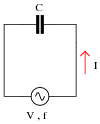Write a single formula solving for capacitance given these three values (V, I, and f).

• #### Question 14

A technician measures voltage across the terminals of a burned-out solenoid valve, in order to check for the presence of dangerous voltage before touching the wire connections. The circuit breaker for this solenoid has been turned off and secured with a lock, but the technician’s digital voltmeter still registers about three and a half volts AC across the solenoid terminals!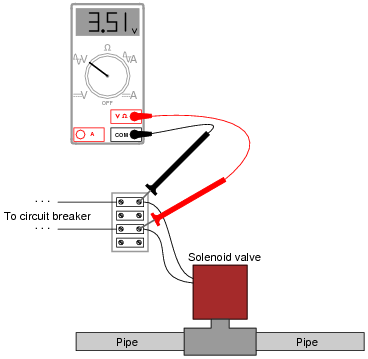Now, three and a half volts AC is not enough voltage to cause any harm, but its presence confuses and worries the technician. Shouldn’t there be 0 volts, with the breaker turned off?

Explain why the technician is able to measure voltage in a circuit that has been “locked out.” Hint: digital voltmeters have extremely high input impedance, typically in excess of 10 MΩ.

• #### Question 15

Audio headphones make highly sensitive voltage detectors for AC signals in the audio frequency range. However, the small speakers inside headphones are quite easily damaged by the application of DC voltage.

Explain how a capacitor could be used as a “filtering” device to allow AC signals through to a pair of headphones, yet block any applied DC voltage, so as to help prevent accidental damage of the headphones while using them as an electrical instrument.

The key to understanding how to answer this question is to recognize what a capacitor “appears as” to AC signals versus DC signals.